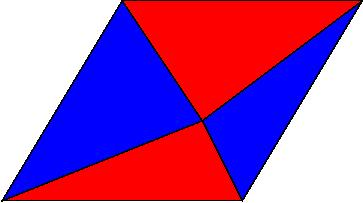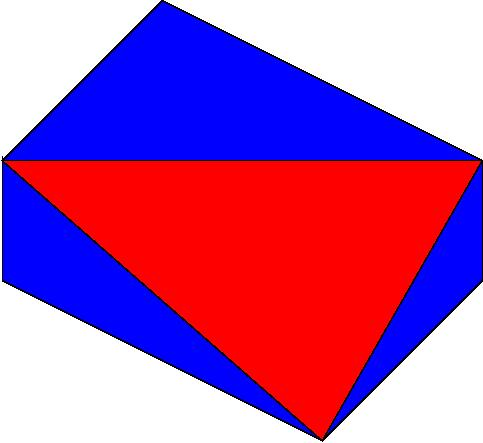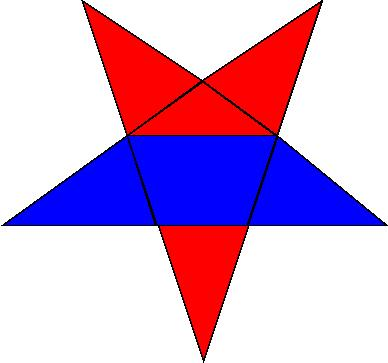Lots of Fun Problems to Prove!!!

Many from "2000 feladat az elemi matematika korebol" by Sandor Roka

Number Theory Examples.

369.  Prove the following divisiblity statements:

•  9| 1033+8
• 6 | 1010+14
• 72 | 1020+ 8

373.  Prove that the following numbers are all composite.

• 106-57
• 10100-7
• 420-1
• 1000...001    (with 1991   0's)
• 111...1111     (with 1989 1's)
• 111...1111      (with 1990 1's)
• 111...121...111  (with the same number of 1's before as after the 2)
• 1!+2!+3!+...+100!
374.  Prove that 347,777,743 is a composite number.

375.  Prove that 49+610+320 is a composite number.

379.  Prove that 989*1001*1007 + 320 is a composite number.

382.  Prove that for any positive integer n, 19*8n + 17 is a composite number.

(There are typo's in the first two-- they should be > n and > sqrt(n) ) I'll Change it asap.)Geometric Proofs

In each of the following problems prove that the area of the blue region is equal to the area of the red.

1284.   Connect any point in the interior of a parallelagram with the four corners.1285.  Connect the midpoints of two opposite sides of a convex quadrilateral to the corners as shown.1288.   In a Hexagon, with opposite sides parallel and of equal length, draw a triangle connecting alternating vertices.1290.   Divide a  regular star as shown.